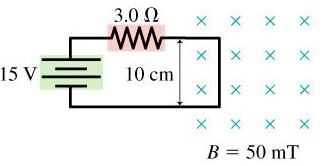# Problem: The right edge of the circuit in the figure extends into a 50 mT uniform magnetic field.What are the magnitude and direction of the net force on the circuit?a) leftb) rightc) upd) down

###### FREE Expert Solution

Current through the conductor:

I = 5.0 A

B = 50 mT

θ (perpendicular) = 90°###### Problem Details

The right edge of the circuit in the figure extends into a 50 mT uniform magnetic field.What are the magnitude and direction of the net force on the circuit?

a) left

b) right

c) up

d) down